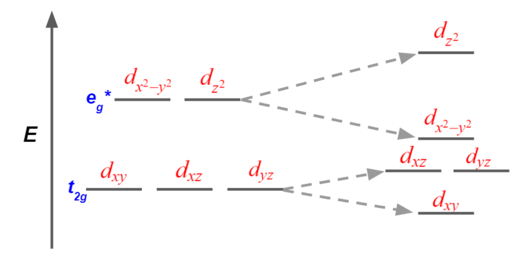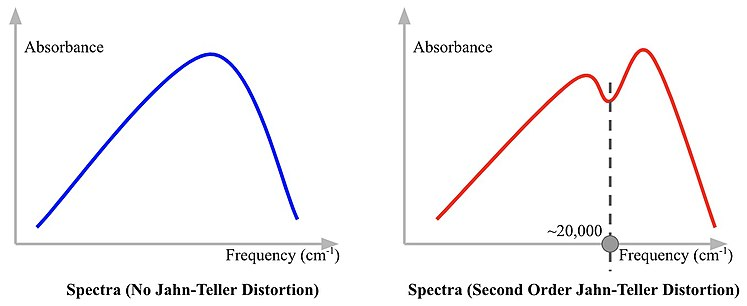# 1.17: Jahn-Teller Distortions

$$\newcommand{\vecs}{\overset { \rightharpoonup} {\mathbf{#1}} }$$ $$\newcommand{\vecd}{\overset{-\!-\!\rightharpoonup}{\vphantom{a}\smash {#1}}}$$$$\newcommand{\id}{\mathrm{id}}$$ $$\newcommand{\Span}{\mathrm{span}}$$ $$\newcommand{\kernel}{\mathrm{null}\,}$$ $$\newcommand{\range}{\mathrm{range}\,}$$ $$\newcommand{\RealPart}{\mathrm{Re}}$$ $$\newcommand{\ImaginaryPart}{\mathrm{Im}}$$ $$\newcommand{\Argument}{\mathrm{Arg}}$$ $$\newcommand{\norm}{\| #1 \|}$$ $$\newcommand{\inner}{\langle #1, #2 \rangle}$$ $$\newcommand{\Span}{\mathrm{span}}$$ $$\newcommand{\id}{\mathrm{id}}$$ $$\newcommand{\Span}{\mathrm{span}}$$ $$\newcommand{\kernel}{\mathrm{null}\,}$$ $$\newcommand{\range}{\mathrm{range}\,}$$ $$\newcommand{\RealPart}{\mathrm{Re}}$$ $$\newcommand{\ImaginaryPart}{\mathrm{Im}}$$ $$\newcommand{\Argument}{\mathrm{Arg}}$$ $$\newcommand{\norm}{\| #1 \|}$$ $$\newcommand{\inner}{\langle #1, #2 \rangle}$$ $$\newcommand{\Span}{\mathrm{span}}$$

## Introduction to Jahn-Teller Distortion

The Jahn–Teller effect occurs because the unequal occupation of orbitals with identical energies is unfavorable. To avoid these unfavorable electronic configurations, molecules distort (lowering their symmetry) to render these orbitals no longer degenerate. The Jahn–Teller distortion, describes the geometrical distortion of molecules and ions that result from certain electron configurations. This distortion is normally observed among octahedral complexes where the two axial bonds can be compressed or elongated to result in a different bond length from those of the equatorial bonds as shown in Figure $$\PageIndex{1}$$.Figure $$\PageIndex{1}$$: The geometric distortion of an octahedral complex

A Brief History of Jahn-Teller Distortion

In 1937, Hermann Jahn and Edward Teller postulated a theorem stating that "stability and degeneracy are not possible simultaneously unless the molecule is a linear one," in regards to its electronic state. This leads to a break in degeneracy which stabilizes the molecule and by consequence, reduces its symmetry.

## Orbital Analysis of Jahn-Teller Distortion

### Elongation Distortion

Elongation as shown in Figure $$\PageIndex{2}$$, is the most common mechanism of distortion. In ideally octahedral complexes that experience Jahn–Teller distortion, the eg* orbitals change more in energy relative to the t2g orbitals.Figure $$\PageIndex{2}$$: The d-orbitals undergo an elongation

When the octahedral complex undergoes an elongation, any of the metal d orbitals that carry a z-directional feature become lower in energy, ligands are easier to bond along z-direction. Geometrically, as the z-direction gets elongated, the repulsion energy and sterical hindrance along z-direction are lower to allow ligands easier to bond.

### Compression Distortion

Compression as shown in Figure $$\PageIndex{3}$$, happens less frequently than the elongation.Figure $$\PageIndex{3}$$: The d-orbitals undergo a compression

When the octahedral complex undergoes a compression, any of the metal d orbitals that carry a z-directional feature become higher in energy, ligands are harder to bond along z-direction. Geometrically, as the z-direction gets compressed, the repulsion energy and sterical hindrance along z-direction are higher, which makes ligands more difficult to bond.

## The Strength of Jahn-Teller Distortion

### General Strength Trend of Jahn-Teller Distortion

For octahedral complexes:

• weak Jahn-Teller effect if t2g is unevenly occupied
• strong Jahn-Teller effect if eg is unevenly occupied

### Table of Jahn-Teller Distortion StrengthFigure $$\PageIndex{4}$$: The table of d-orbitals Jahn-Teller distortion strength w = weak Jahn-Teller effect expected; s = strong Jahn-Teller effect expected; - = no Jahn-Teller distortion

## Jahn-Teller Distortion and Spectra

### Spectra of the First-order Jahn-Teller Distortion

In the first-order Jahn-Teller distortion, two electron transitions can be observed. This phenomenon can lead to a “double-hump” result on the absorption spectra as shown in Figure $$\PageIndex{5}$$ and $$\PageIndex{6}$$.Figure $$\PageIndex{5}$$: The absorption spectra of a first-order Jahn-Teller distortionFigure $$\PageIndex{6}$$: The electron transition in non-distorted (left) and distorted (right) orbitals

### The Second-order Jahn-Teller Distortion

#### A brief introduction to the Second-order Jahn-Teller Distortion

The second order Jahn-Teller distortion occurs when the excited state of the transition metal has unequal occupation of d-orbitals with identical energies. The ground state of the transition metal may not have unequal occupation of degenerate orbitals, therefore, the second order Jahn-Teller distortion is also called Pseudo Jahn-Teller distortion as shown in Figure $$\PageIndex{7}$$.Figure $$\PageIndex{7}$$: Energy profiles in a Pseudo Jahn-Teller distortion versus the Jahn-Teller distortion

#### Spectra of the Second-order Jahn-Teller Distortion

Since the second order Jahn-Teller distortion occurs in excited state, the trough on the spectra likely located at higher frequency, thus higher in energy, as shown in Figure $$\PageIndex{8}$$.Figure $$\PageIndex{8}$$: The absorption spectra of a second-order Jahn-Teller distortion

Figure $$\PageIndex{8}$$.

#### References

1. ↑ Libretexts. “Jahn-Teller Distortions.” Chemistry LibreTexts, Libretexts, 23 Apr. 2019,
2. ↑ Libretexts. “5.7: Jahn-Teller Effect.” Chemistry LibreTexts, Libretexts, 23 Apr. 2019
3. ↑ Libretexts. “Jahn-Teller Distortions.” Chemistry LibreTexts, Libretexts, 23 Apr. 2019
4. ↑ “Jahn–Teller Effect.” Wikipedia, Wikimedia Foundation, 7 Mar. 2019
5. ↑ Libretexts. “Jahn-Teller Distortions.” Chemistry LibreTexts, Libretexts, 23 Apr. 2019
6. ↑ Miessler, Gary L., et al. Inorganic Chemistry. Pearson, 2014.
7. ↑ Bersuker, Isaac B. (9 January 2013). "Pseudo-Jahn–Teller Effect—A Two-State Paradigm in Formation, Deformation, and Transformation of Molecular Systems and Solids". Chemical Reviews. American Chemical Society (ACS). 113 (3): 1351–1390. doi:10.1021/cr300279n. ISSN 0009-2665.
8. ↑ “File:Jahn-Teller and Pseudo Jahn-Teller Effects.svg.” File:Jahn-Teller and Pseudo Jahn-Teller Effects.svg - Wikimedia Commons

1.17: Jahn-Teller Distortions is shared under a CC BY-SA license and was authored, remixed, and/or curated by LibreTexts.